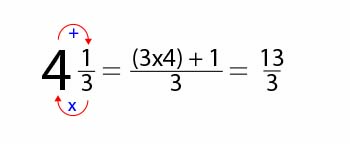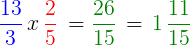# Multiplying Compound Fractions

Instructor: Nicole Huppenthal

Nicole has taught at both the elementary and high school levels, specializing in gifted education, elementary education, and technology.

This lesson keeps you moving along the path of mastering fractions. We'll unwrap the steps to multiplying compound fractions, also known as mixed numbers.

## Fraction Refresher

Whether you like to bake treats in the kitchen, using 1/2 teaspoon salt and 1/8 teaspoon baking powder or work with tools to build something new, cutting 3/4'' off a piece of wood, you know that fractions are everywhere. So, it's helpful to know how to work with them to get our projects done.

We already know that fractions are an equal part of a whole, and when you are multiplying fractions, you are simply adding together groups of the same number. Multiplication is just a faster way to get there. So let's get started!

## Compound Fractions

A compound fraction, also known as a mixed number, is a combination of a fraction and a whole number. You will remember that when you multiply fractions, you go straight across, multiplying the numerators, numbers on top, and then multiplying the denominators, the numbers on the bottom. In order to multiply compound fractions, we first have to convert the compound fraction into an improper fraction, which is a fraction whose numerator is larger than its denominator. Let's take a look.

### Converting Compound Fractions

We take the whole number in front of the fraction and multiply it by the denominator. In our example of 4 1/3, we will multiply 4 (our whole number) x 3 (the denominator of our fraction). We need to know how many total parts we have.

We take this product, 12 (4 x 3 = 12) and add it to the numerator that we already have, in this example 1 for the total, 12 + 1 = 13. We still leave this number over the same denominator, 3. So, we now have an improper fraction (numerator is greater than the denominator) of 13/3.

If you are multiplying two compound fractions, you will need to do this to each one.### Multiplying Compound Fractions

We have finally arrived at the heart of this lesson: Multiplying compound fractions.

Now that we have converted our compound fraction into an improper fraction, we can easily multiply it with another fraction. This is a skill you already have, but let's just try one example before moving on:

1. Multiply the numerators straight across.
2. Multiply the denominators straight across.
3. Convert - change an improper fraction back to a mixed number.To unlock this lesson you must be a Study.com Member.

### Register to view this lesson

Are you a student or a teacher?

#### See for yourself why 30 million people use Study.com

##### Become a Study.com member and start learning now.
Back
What teachers are saying about Study.com

### Earning College Credit

Did you know… We have over 200 college courses that prepare you to earn credit by exam that is accepted by over 1,500 colleges and universities. You can test out of the first two years of college and save thousands off your degree. Anyone can earn credit-by-exam regardless of age or education level.